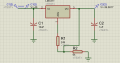# Converting voltages using LM337

#### Oussama Zaidi

Joined Mar 1, 2016
53
Hi
I have 2 questions:
Is it possible to generate -35V from LM337 regulator with -12V in input??
The voltage output from LM337 is supposed to follow this equation, -Vout=-1.25(1+R2/R1)+Iadj.R2 but in ISIS simulation the output value is always the same as the input, I don't understand why, for example in the picture above output value is supposed to be -32V according to the resistances values but it is -39V because the input is -40V, the same for any other voltage.#### Alec_t

Joined Sep 17, 2013
13,783
You have R2 in the wrong place. It should connect ADJ to ground.

#### AnalogKid

Joined Aug 1, 2013
10,573
Is it possible to generate -35V from LM337 regulator with -12V in input??
No.

Besides the schematic error, that is not how a linear regulator functions. Basically, it acts like an automatic attenuator. So the equation is correct as long as the output is less than the input. With a -12 V input, the maximum output is around -10 V. This is because the device needs a minimum of 2 volts or so (the exact number is on the datasheet) for it to function.

Some switching regulator circuits can do what you want, but linear regulators sannot.

ak

#### Oussama Zaidi

Joined Mar 1, 2016
53
No.

Besides the schematic error, that is not how a linear regulator functions. Basically, it acts like an automatic attenuator. So the equation is correct as long as the output is less than the input. With a -12 V input, the maximum output is around -10 V. This is because the device needs a minimum of 2 volts or so (the exact number is on the datasheet) for it to function.

Some switching regulator circuits can do what you want, but linear regulators sannot.

ak
@AnalogKid Can you give me some names of switching regulators can do the job, I do my research but I didn't found any, just anything can give lower than -30V.

#### absf

Joined Dec 29, 2010
1,968